# (extended) Ceva's trisectrix

## sexticThis sextic 1) is a botanic curve.Giovanni Ceva (1648-1734), an Italian mathematician and engineer, studied the curve for b=2. This was in origin Ceva's trisectrix. The curve can be written as r = sin3φ/sinφ. The trisectrix can be extended to other values for b. Ceva's trisectrix can be used for the trisection of an angle, as follows (see picture to the right). Let there be a circle C with center O. Draw a line through O which cuts C in P. Construct a point Q on the x-axis so that OP = PQ. Then Ceva's trisectrix is the collection of points M for which: M lies on the line through OP MP = PQ Now the angle OQM is three times the angle QOM. For b = 1/2, the curve is called the peanut curve. For b = 1, the curve is called the double egg. Its equation can also be written as: r = cos2φ. For b = 2, the curve is called the bow tie. Some examples: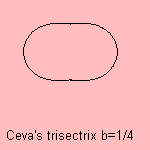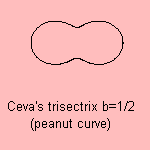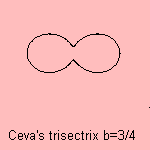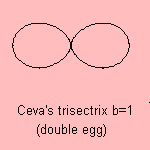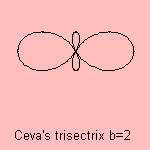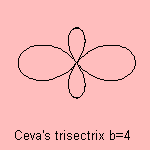For large values of parameter b, the curve approximates the quadrifolium (rhodonea c=2).     notes 1) Cartesian equation:  (x2 + y2)3 = ((b+1)x2 - (b-1)y2)2